# Physics - Faraday's Law of Electro - Magnetic Introduction

Faraday's Law of Electro - Magnetic Introduction

The induced e. m. f is directly proportional the rate of change of magnetic flux  that is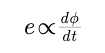Where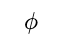is the flux linkage for one turn.

If the coil contains N turns an e. m. f appears in every turn and all these e. m. f's to be added.

If the coil has N turrns then,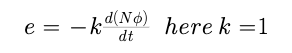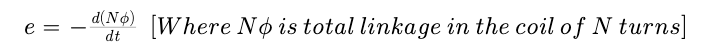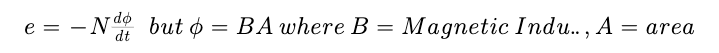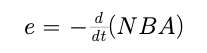Negative Sign indicates that the induced emf opposes any change according to Lenz's law .

It is also called as Neumann's las

Post By : Ashish Maurya 17 May, 2020 413 views Physics